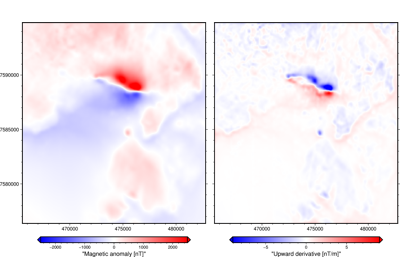# harmonica.derivative_upward¶

harmonica.derivative_upward(grid, order=1)[source]

Calculate the derivative of a potential field grid in the upward direction

Compute the spatial derivative in the upward direction of regular gridded data using frequency domain calculations through Fast Fourier Transform.

Parameters
• grid (`xarray.DataArray`) – A two dimensional `xarray.DataArray` whose coordinates are evenly spaced (regular grid). Its dimensions should be in the following order: northing, easting. Its coordinates should be defined in the same units.

• order (int) – The order of the derivative. Default to 1.

Returns

derivative (`xarray.DataArray`) – A `xarray.DataArray` with the upward derivatives of the passed `grid`. Its units are the same units of the `grid` per units of its coordinates.

References

[Blakely1995]

## Examples using `harmonica.derivative_upward`¶